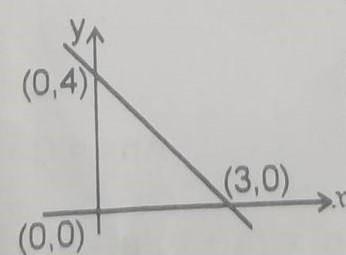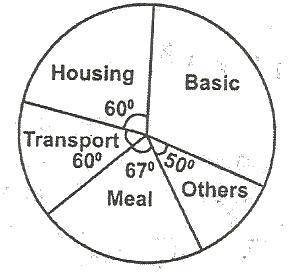# Mathematics 2020 Past Questions | JAMB

Study the following Mathematics past questions and answers for JAMBWAEC  NECO and Post-JAMB. Get prepared with official past questions and answers for upcoming examinations.

16. A crate of soft drinks contains 10 bottle of Coca-Cola 8 of Fanta and 6 of Sprite. If one bottle is selected at random, what is the probability that it is Not a Coca-Cola bottle?

• A. 5/12
• B. 1/3
• C. 3/4
• D. 7/12

17.  In this figure, PQ = PR = PS and SRT = 68o. Find QPS• A. 136o
• B. 124o
• C. 112o
• D. 68o

18. Find the gradient of the line passing through the points (-2, 0) and (0, 4)

• A. 2
• B. -4
• C. -2
• D. 4

19. Find the equation of the line through the points (5, 7) parallel to the line 7x + 5y = 12.

• A. 5x + 7y = 120
• B. 7x + 5y = 70
• C. x + y = 7
• D. 15x + 17y = 90

20.  If N225.00 yields N27.00 in x years simple interest at the rate of 4% per annum, find x.

• A. 3
• B. 4
• C. 12
• D. 17

21.  Calculate the standard deviation of the following data: 7, 8, 9, 10, 11, 12, 13

• A. 2
• B. 4
• C. 12
• D. 17

22.  A number is selected at random between 20 and 30, both numbers inclusive. Find the probability that the number is a prime.

• A. 2/11
• B. 5/11
• C. 6/11
• D. 8/11

23. The equation of the line in the graph is• A. 3y = 3x + 12
• B. 3y = 3x + 12
• C. 3y = -4x + 12
• D. 3y = -4x + 9

24.  Express the product of 0.0014 and 0.011 in standard form

• A. 1.54 x 10−2
• B. 1.54 x 10−3
• C. 1.54 x 10−2
• D. 1.54 x 10−5

25. A school boy lying on the ground 30m away from the foot of a water tank towel observes that the angle of elevation of the top of the tank is 60o. Calculate the height of the tank.

• A. 60√3m
• B. 30√3m
• C. 20√3m
• D. 10√3m

26. Find the simple interest rate percent annum at which N1000 accumulates to N1240 in 3 years.

• A. 6%
• B. 8%
• C. 10%
• D. 12%

27.  The pie chart shows the income of a civil servant in month. If his monthly income is N6,000. Find his monthly basic salary.• A. N2,050
• B. N2600
• C. N3,100
• D. N3,450

28. If x is positive real number, find the range of values for which 1/3x + 1/2> 1/4x

• A. x > -1/6
• B. x > 0
• C. 0 < x < 6
• D. 0 < x <1/6

29.  Find the value of x if = √2 / x +  √2 = 1/  x – √2

• A. 3√2 + 4
• B. 3√2 – 4
• C. 3 – 2√2
• D. 4 + 2√2

30. A binary operation x is defined by a x b = ab. If a x 2 = 2 – a, find the possible values of a?

• A. 1, -2
• B. 2, -1
• C. 2, -2
• D. 1, -1

## All Subjects

Subscribe
Notify of